Type
Quiz
Book Title
Fundamentals of Corporate Finance Standard Edition 9th Edition
ISBN 13
978-0073382395

### 978-0073382395 Chapter 9 Questions and Problems 1-15

April 3, 2019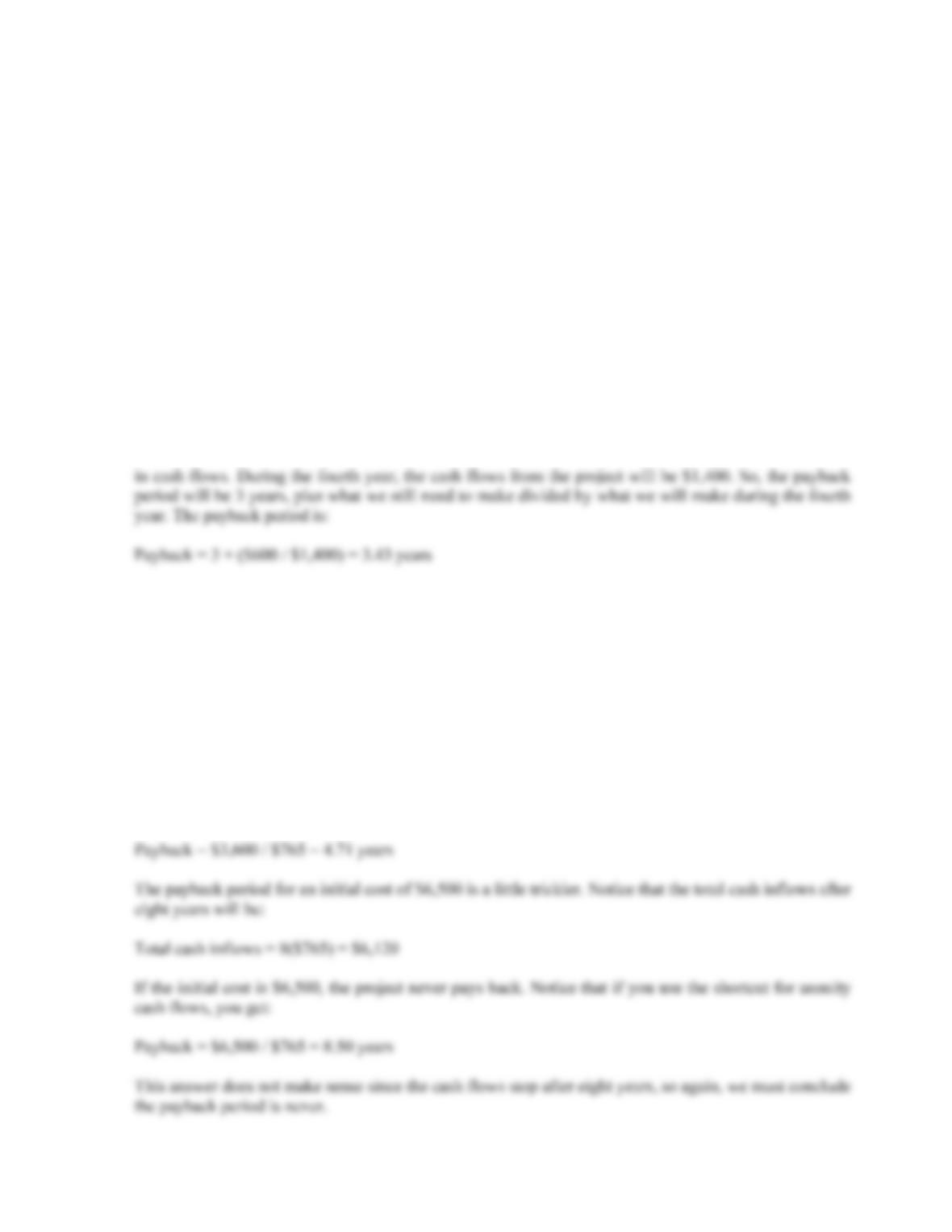CHAPTER 9 B-157
Solutions to Questions and Problems
NOTE: All end of chapter problems were solved using a spreadsheet. Many problems require multiple steps.
Due to space and readability constraints, when these intermediate steps are included in this solutions
manual, rounding may appear to have occurred. However, the final answer for each problem is found
without rounding during any step in the problem.
Basic
1. To calculate the payback period, we need to find the time that the project has recovered its initial
investment. After three years, the project has created:
\$1,600 + 1,900 + 2,300 = \$5,800
in cash flows. The project still needs to create another:
\$6,400 – 5,800 = \$600
2. To calculate the payback period, we need to find the time that the project has recovered its initial
investment. The cash flows in this problem are an annuity, so the calculation is simpler. If the initial
cost is \$2,400, the payback period is:
Payback = 3 + (\$105 / \$765) = 3.14 years
There is a shortcut to calculate the future cash flows are an annuity. Just divide the initial cost by the
annual cash flow. For the \$2,400 cost, the payback period is:
Payback = \$2,400 / \$765 = 3.14 years
For an initial cost of \$3,600, the payback period is: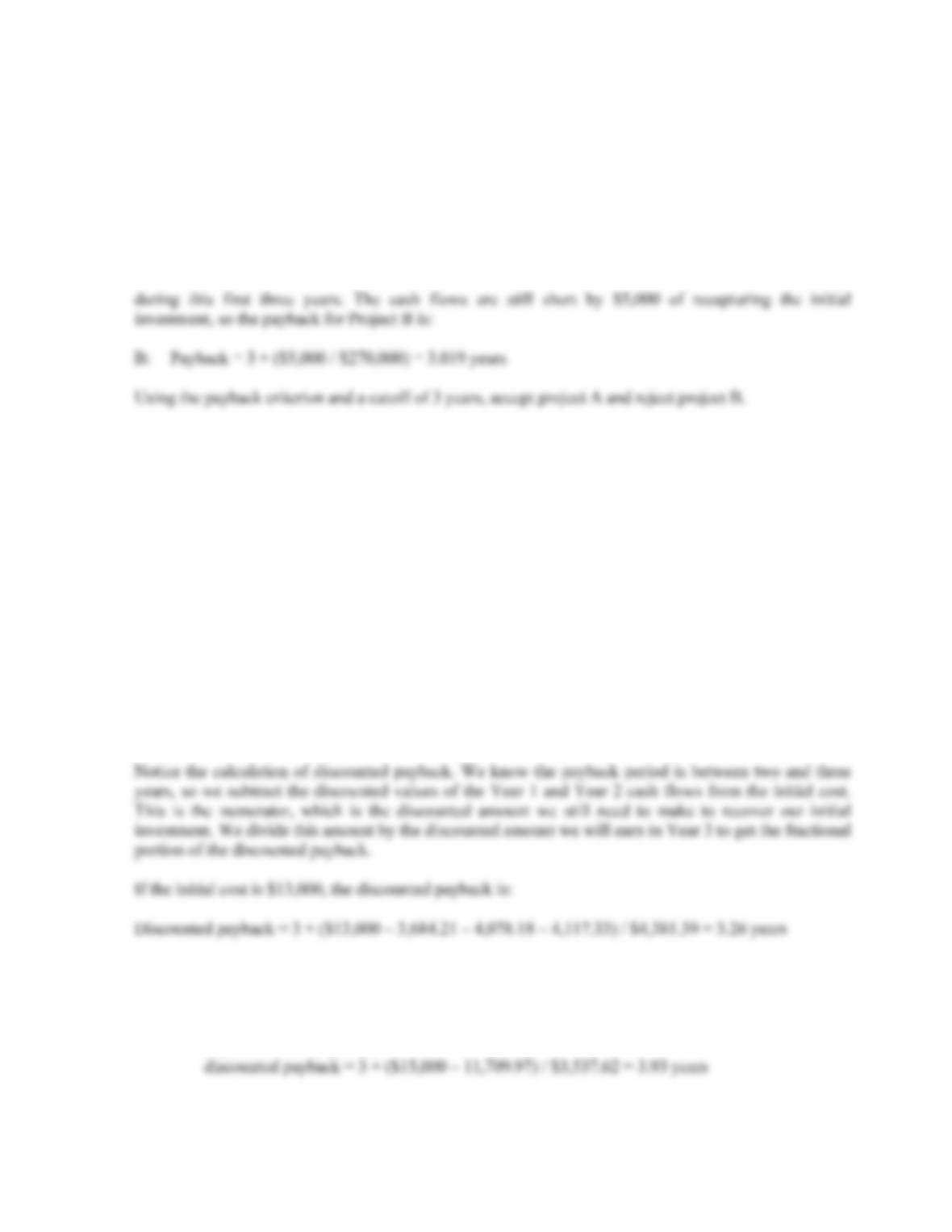B-158 SOLUTIONS
3. Project A has cash flows of \$19,000 in Year 1, so the cash flows are short by \$21,000 of recapturing the
initial investment, so the payback for Project A is:
Payback = 1 + (\$21,000 / \$25,000) = 1.84 years
Project B has cash flows of:
Cash flows = \$14,000 + 17,000 + 24,000 = \$55,000
4. When we use discounted payback, we need to find the value of all cash flows today. The value today of
the project cash flows for the first four years is:
Value today of Year 1 cash flow = \$4,200/1.14 = \$3,684.21
Value today of Year 2 cash flow = \$5,300/1.142 = \$4,078.18
Value today of Year 3 cash flow = \$6,100/1.143 = \$4,117.33
Value today of Year 4 cash flow = \$7,400/1.144 = \$4,381.39
To find the discounted payback, we use these values to find the payback period. The discounted first
year cash flow is \$3,684.21, so the discounted payback for a \$7,000 initial cost is:
Discounted payback = 1 + (\$7,000 – 3,684.21)/\$4,078.18 = 1.81 years
For an initial cost of \$10,000, the discounted payback is:
Discounted payback = 2 + (\$10,000 – 3,684.21 – 4,078.18)/\$4,117.33 = 2.54 years
5. R = 0%: 3 + (\$2,100 / \$4,300) = 3.49 years
discounted payback = regular payback = 3.49 years
R = 5%: \$4,300/1.05 + \$4,300/1.052 + \$4,300/1.053 = \$11,709.97
\$4,300/1.054 = \$3,537.62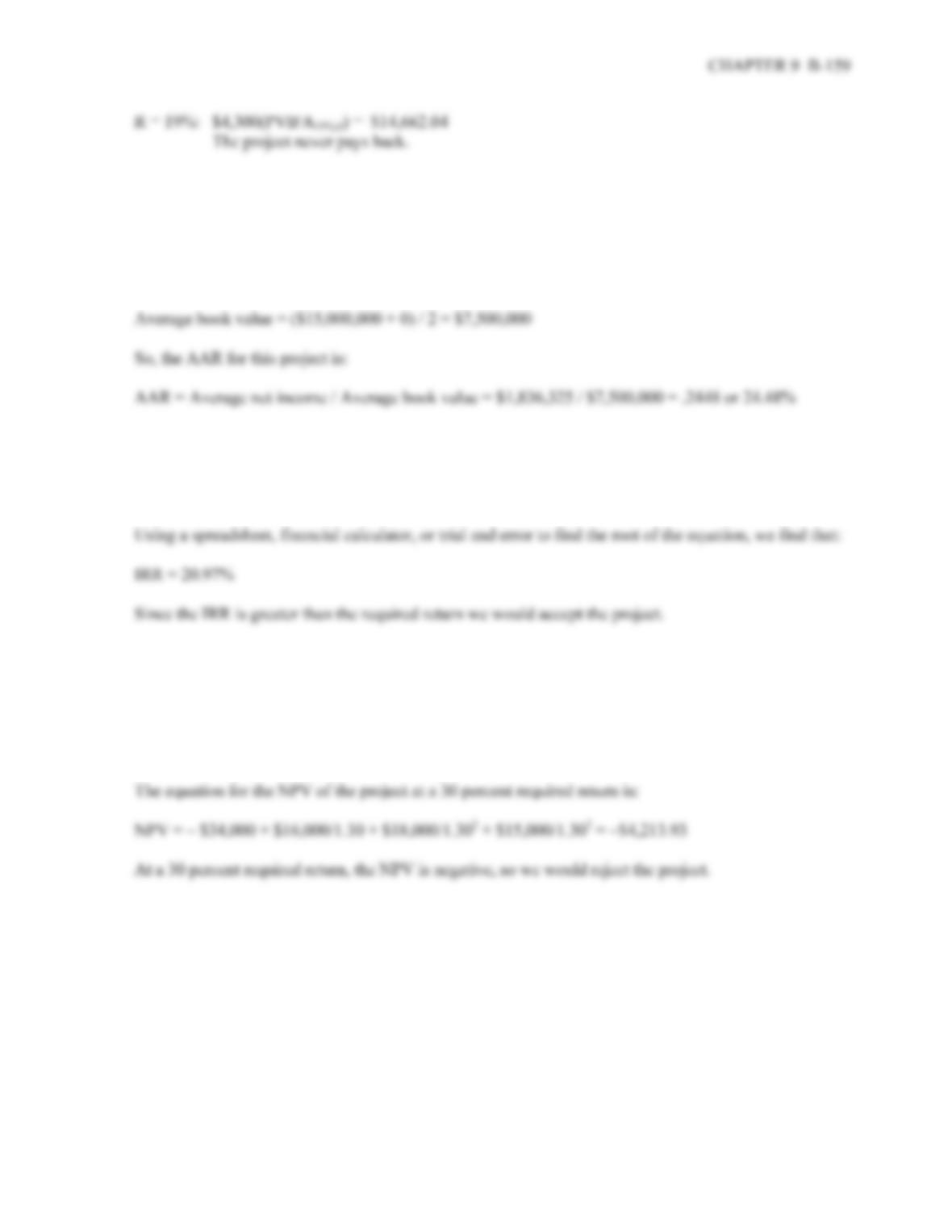6. Our definition of AAR is the average net income divided by the average book value. The average net
income for this project is:
Average net income = (\$1,938,200 + 2,201,600 + 1,876,000 + 1,329,500) / 4 = \$1,836,325
And the average book value is:
7. The IRR is the interest rate that makes the NPV of the project equal to zero. So, the equation that defines the
IRR for this project is:
0 = – \$34,000 + \$16,000/(1+IRR) + \$18,000/(1+IRR)2 + \$15,000/(1+IRR)3
8. The NPV of a project is the PV of the outflows minus the PV of the inflows. The equation for the NPV
of this project at an 11 percent required return is:
NPV = – \$34,000 + \$16,000/1.11 + \$18,000/1.112 + \$15,000/1.113 = \$5,991.49
At an 11 percent required return, the NPV is positive, so we would accept the project.
9. The NPV of a project is the PV of the outflows minus the PV of the inflows. Since the cash inflows are
an annuity, the equation for the NPV of this project at an 8 percent required return is:
NPV = –\$138,000 + \$28,500(PVIFA8%, 9) = \$40,036.31
At an 8 percent required return, the NPV is positive, so we would accept the project.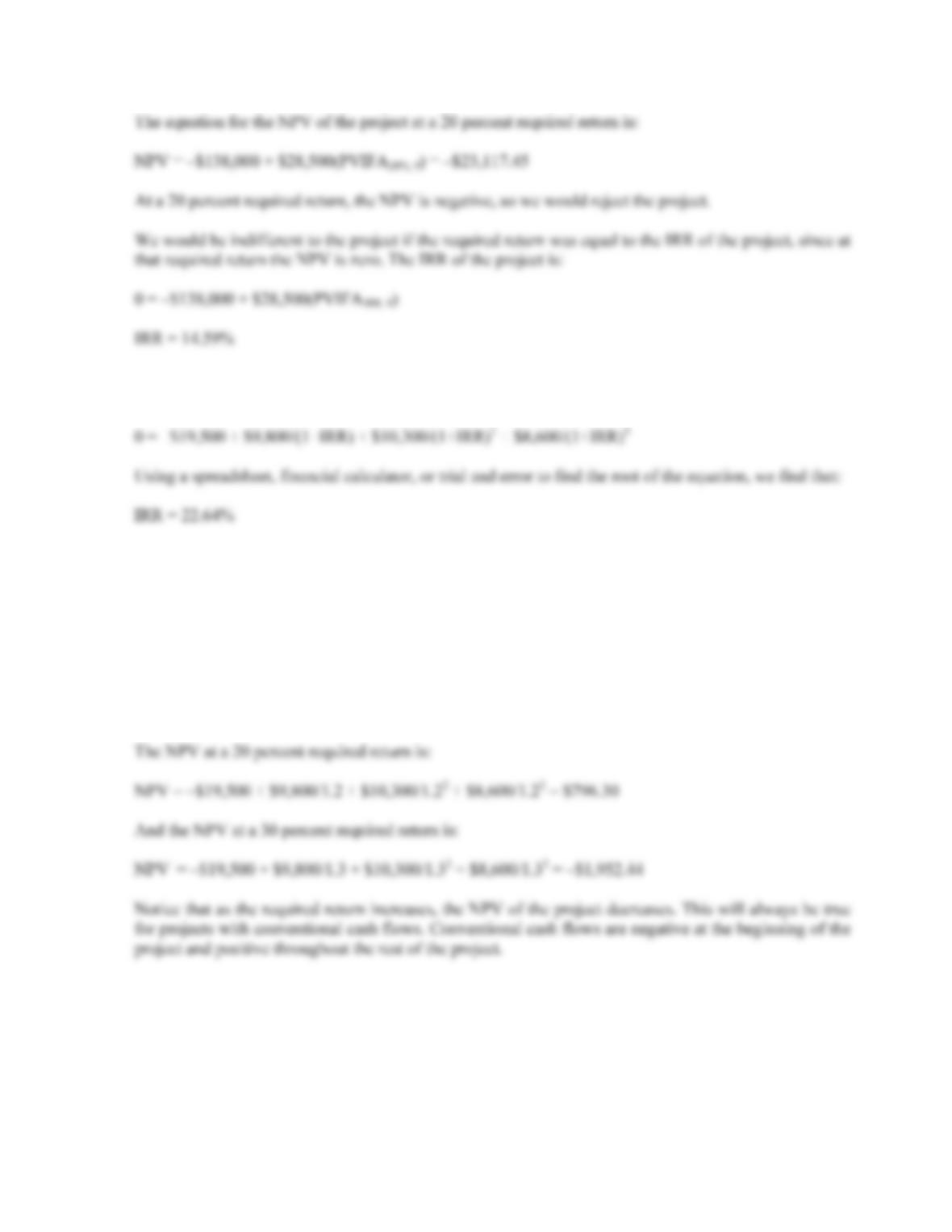B-160 SOLUTIONS
10. The IRR is the interest rate that makes the NPV of the project equal to zero. So, the equation that defines the
IRR for this project is:
11. The NPV of a project is the PV of the outflows minus the PV of the inflows. At a zero discount rate
(and only at a zero discount rate), the cash flows can be added together across time. So, the NPV of the
project at a zero percent required return is:
NPV = –\$19,500 + 9,800 + 10,300 + 8,600 = \$9,200
The NPV at a 10 percent required return is:
NPV = –\$19,500 + \$9,800/1.1 + \$10,300/1.12 + \$8,600/1.13 = \$4,382.79CHAPTER 9 B-161
12. a. The IRR is the interest rate that makes the NPV of the project equal to zero. The equation for the IRR of
Project A is:
0 = –\$43,000 + \$23,000/(1+IRR) + \$17,900/(1+IRR)2 + \$12,400/(1+IRR)3 + \$9,400/(1+IRR)4
Using a spreadsheet, financial calculator, or trial and error to find the root of the equation, we find that:
IRR = 20.44%
b. The NPV of Project A is:
NPVA = –\$43,000 + \$23,000/1.11+ \$17,900/1.112 + \$12,400/1.113 + \$9,400/1.114
NPVA = \$7,507.61
c. To find the crossover rate, we subtract the cash flows from one project from the cash flows of the
other project. Here, we will subtract the cash flows for Project B from the cash flows of Project A.
Once we find these differential cash flows, we find the IRR. The equation for the crossover rate is:
Crossover rate: 0 = \$16,000/(1+R) + \$4,100/(1+R)2 – \$11,600/(1+R)3 – \$16,600/(1+R)4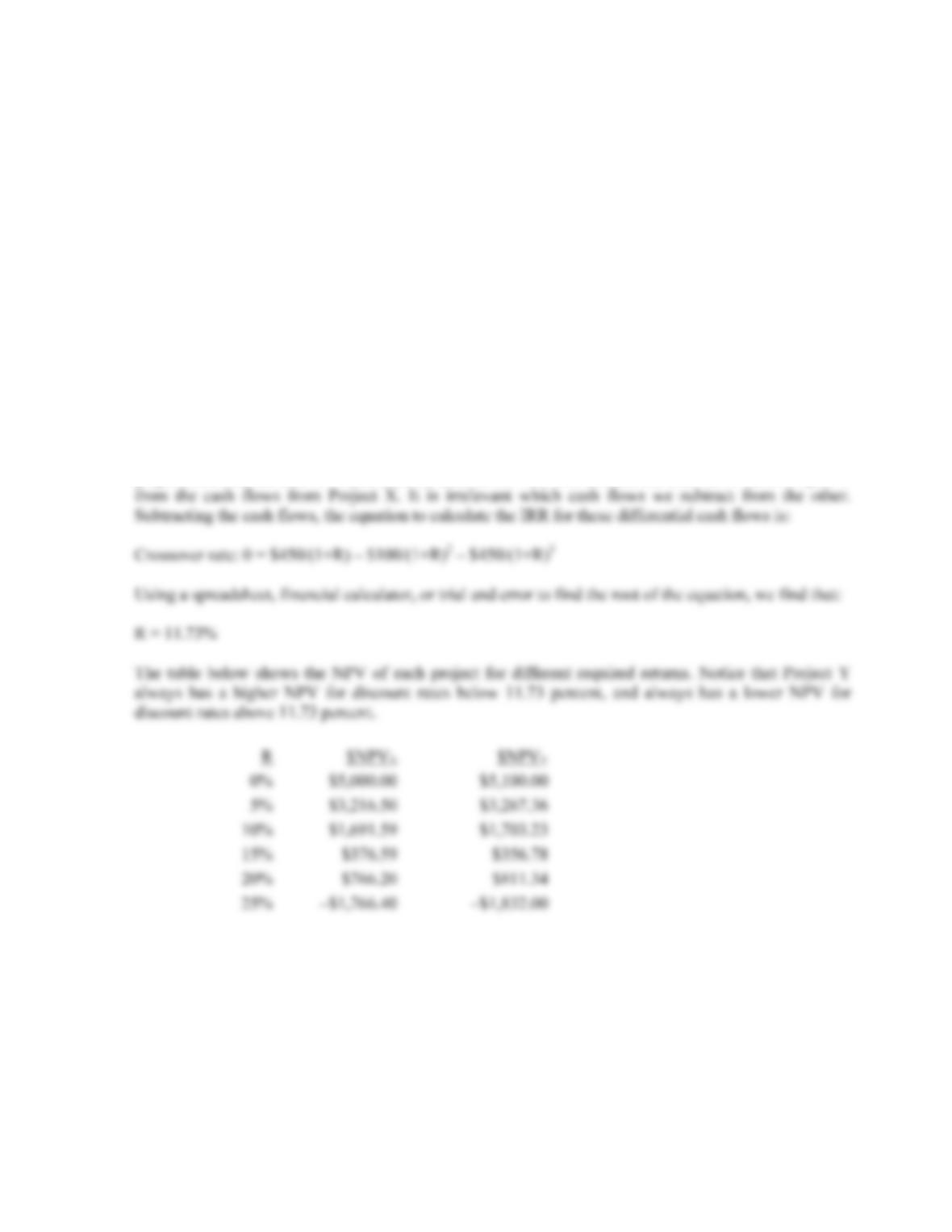B-162 SOLUTIONS
13. The IRR is the interest rate that makes the NPV of the project equal to zero. The equation to calculate
the IRR of Project X is:
0 = –\$15,000 + \$8,150/(1+IRR) + \$5,050/(1+IRR)2 + \$6,800/(1+IRR)3
Using a spreadsheet, financial calculator, or trial and error to find the root of the equation, we find that:
IRR = 16.57%
For Project Y, the equation to find the IRR is:
0 = –\$15,000 + \$7,700/(1+IRR) + \$5,150/(1+IRR)2 + \$7,250/(1+IRR)3
Using a spreadsheet, financial calculator, or trial and error to find the root of the equation, we find that:
IRR = 16.45%
To find the crossover rate, we subtract the cash flows from one project from the cash flows of the other
project, and find the IRR of the differential cash flows. We will subtract the cash flows from Project Y
14. a. The equation for the NPV of the project is:
NPV = –\$45,000,000 + \$78,000,000/1.1 – \$14,000,000/1.12 = \$13,482,142.86
The NPV is greater than 0, so we would accept the project.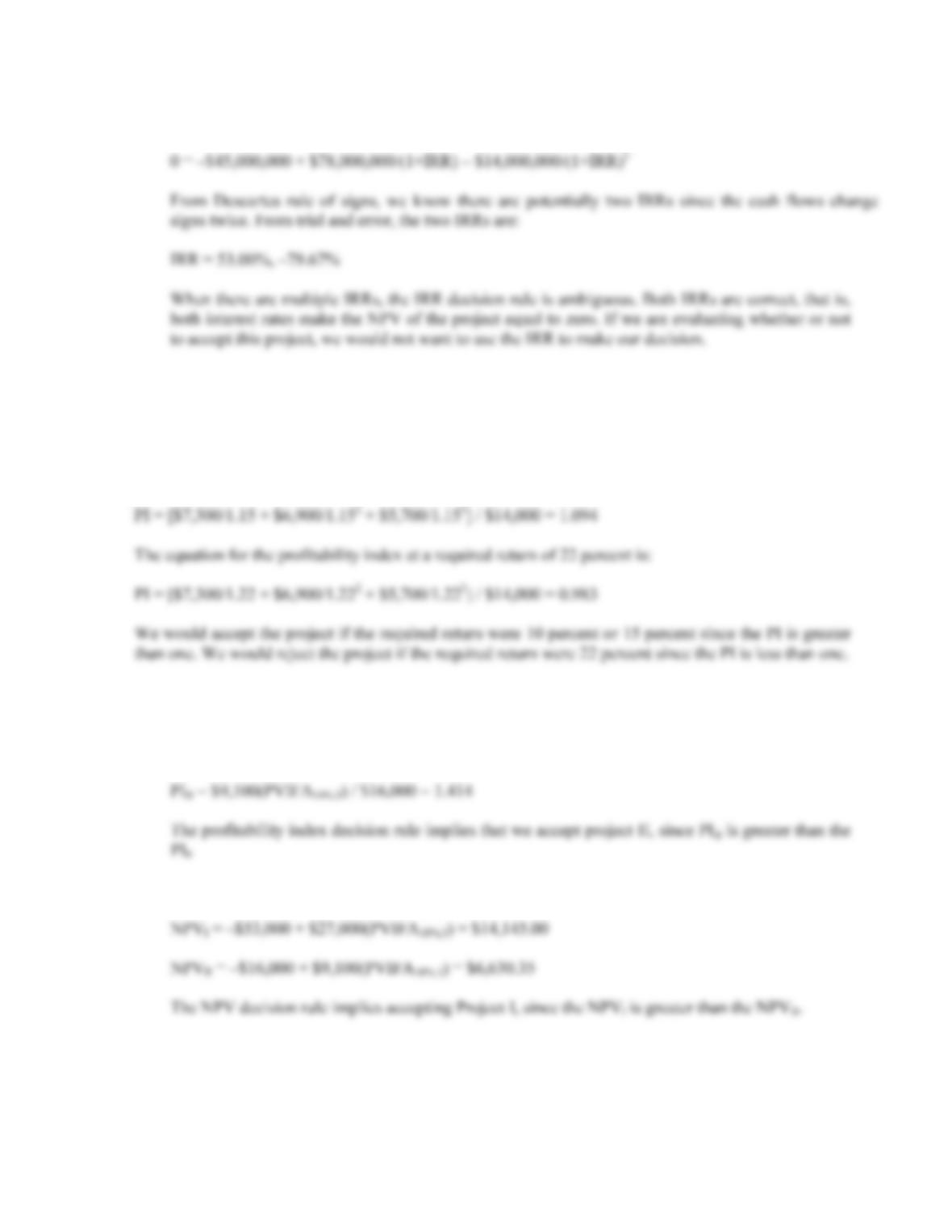CHAPTER 9 B-163
b. The equation for the IRR of the project is:
15. The profitability index is defined as the PV of the cash inflows divided by the PV of the cash outflows.
The equation for the profitability index at a required return of 10 percent is:
PI = [\$7,300/1.1 + \$6,900/1.12 + \$5,700/1.13] / \$14,000 = 1.187
The equation for the profitability index at a required return of 15 percent is:
16. a. The profitability index is the PV of the future cash flows divided by the initial investment. The cash
flows for both projects are an annuity, so:
PII = \$27,000(PVIFA10%,3 ) / \$53,000 = 1.267
b. The NPV of each project is: